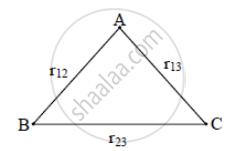# Define electrostatic potential at a point. Write its S.I. unit. Three point charges q1, q2 and q3 are kept respectively at points A, B, and C as shown in the figure, - Physics

Define electrostatic potential at a point. Write its S.I. unit. Three-point charges q1, q2 and q3 are kept respectively at points A, B, and C as shown in the figure, Derive the expression for the electrostatic potential energy of the system.#### Solution

The electrostatic potential (V ) at any point in a region with electrostatic field is the work done in bringing a unit positive charge (without acceleration) from infinity to that point. Its S.I. unit is Volt. The potential energy of a system of three charges q1, q2, and q3 located at vec"r"_1,vec"r"_2,vec"r"_3,respectively. To bring q1 first from infinity to vecr_1, no work is required. Next, we bring q2 from infinity to vec r_2,. As before, work done in this step is

q_2"V"_1(vecr_2,)= (1)/(4piepsilon_0) (q_1q_2)/"r"_12   ...(1)

The charges q1and q2 produce a potential, which at any point P is given by

"V"_(1,2) = (1)/(4piepsilon_0)(q_1/"r"_(1"P") + q_2/"r"_(2"P"))   .......(2)

Work done next in bringing q3 from infinity to the point vec"r"_3  "is q3 times V1, 2 at"

q_3"V"_(1,2) (vec"r"_3) = (1)/(4piepsilon_0)((q_1q_2)/"r"_12+ (q_2q_3)/"r"_23)    ...(3)

The total work done in assembling the charges at the given locations is obtained by adding the work done in different steps [Eq. (1) and Eq. (3)] and gets stored in the form of potential energy.

"U" = (1)/(4piepsilon_0) ((q_1q_2)/"r"_12 + (q_1q_3)/"r"_13 + (q_2q_3)/"r"_23).

Concept: Electrostatics of Conductors
Is there an error in this question or solution?